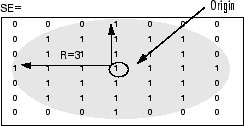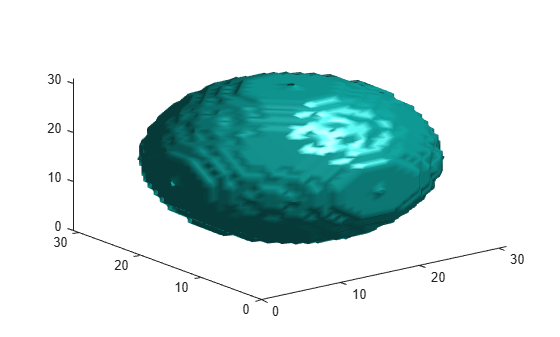# strel

Morphological structuring element

## Description

A `strel` object represents a flat morphological structuring element, which is an essential part of morphological dilation and erosion operations.

A flat structuring element is a binary valued neighborhood, either 2-D or multidimensional, in which the `true` pixels are included in the morphological computation, and the `false` pixels are not. The center pixel of the structuring element, called the origin, identifies the pixel in the image being processed. Use the `strel` function (described below) to create a flat structuring element. You can use flat structuring elements with both binary and grayscale images. The following figure illustrates a flat structuring element.To create a nonflat structuring element, use `offsetstrel`.

## Creation

### Syntax

``SE = strel(nhood)``
``SE = strel("diamond",r)``
``SE = strel("disk",r)``
``SE = strel("disk",r,n)``
``SE = strel("octagon",r)``
``SE = strel("line",len,deg)``
``SE = strel("rectangle",[m n])``
``SE = strel("square",w)``
``SE = strel("cube",w)``
``SE = strel("cuboid",[m n p])``
``SE = strel("sphere",r)``

### Description

#### Arbitrary Neighborhood Shape

````SE = strel(nhood)` creates a flat structuring element with specified neighborhood `nhood`.```

#### 2-D Geometric Neighborhood Shapes

````SE = strel("diamond",r)` creates a diamond-shaped structuring element, where `r` specifies the distance from the structuring element origin to the points of the diamond.```

example

````SE = strel("disk",r)` creates a disk-shaped structuring element, where `r` specifies the radius.```
````SE = strel("disk",r,n)` creates a disk-shaped structuring element, where `r` specifies the radius and `n` specifies the number of line structuring elements used to approximate the disk shape. Morphological operations run much faster when the structuring element uses approximations.```
````SE = strel("octagon",r)` creates a octagonal structuring element, where `r` specifies the distance from the structuring element origin to the sides of the octagon, as measured along the horizontal and vertical axes. `r` must be a nonnegative multiple of 3.```

example

````SE = strel("line",len,deg)` creates a linear structuring element that is symmetric with respect to the neighborhood center, with approximate length `len` and angle `deg`.```
````SE = strel("rectangle",[m n])` creates a rectangular structuring element of size ```[m n]```.```

example

````SE = strel("square",w)` creates a square structuring element whose width is `w` pixels.```

#### 3-D Geometric Neighborhood Shapes

````SE = strel("cube",w)` creates a 3-D cubic structuring element whose width is `w` pixels.```
````SE = strel("cuboid",[m n p])` creates a 3-D cuboidal structuring element of size m-by-n-by-p pixels. ```

example

````SE = strel("sphere",r)` creates a 3-D spherical structuring element whose radius is `r` pixels.```
Compatibility

The following syntaxes still work, but `offsetstrel` is the preferred way to create these nonflat structuring element shapes:

• `SE = strel("arbitrary",nhood,h)`, where `h` is a matrix of the same size as `nhood` containing the height values associated with each nonzero element of `nhood`.

• `SE = strel("ball",r,h,n)`

### Input Arguments

expand all

Neighborhood, specified as numeric array of any dimension. All nonzero pixels of `nhood` belong to the neighborhood for the morphological operation. The center (or origin) of `nhood` is its center element, given by `floor((size(nhood) + 1)/2)`.

Data Types: `single` | `double` | `int8` | `int16` | `int32` | `int64` | `uint8` | `uint16` | `uint32` | `uint64` | `logical`

Radius of the structuring element, specified as a positive integer.

• For the disk shape, `r` is the distance from the origin to the edge of the disk.

• For the diamond shape, `r` is the distance from the structuring element origin to the points of the diamond.

• For the octagon shape, `r` is the distance from the structuring element origin to the sides of the octagon, as measured along the horizontal and vertical axes. `r` must be a multiple of 3.

• For the sphere shape, `r` is the distance from the origin to the edge of the sphere.

Data Types: `double`

Number of periodic line structuring elements used to approximate shape, specified as `0`, `4`, `6`, or `8`. Morphological operations using disk approximations run much faster when the structuring element uses approximations (`n` > 0).

Value of nBehavior
`n` > 0`strel` uses a sequence of `n` periodic line-shaped structuring elements to approximate the shape. Sometimes `strel` must use two extra line structuring elements in the approximation, in which case the actual number of decomposed structuring elements is `n+2`.
`n` = 0`strel` does not use any approximation. The structuring element members comprise all pixels whose centers are no greater than `r` away from the origin.

Data Types: `double`

Length of linear structuring element, specified as a positive number. `len` is approximately the distance between the centers of the structuring element members at opposite ends of the line.

Data Types: `double`

Angle of linear structuring element, in degrees, specified as numeric scalar. The angle is measured in a counterclockwise direction from the horizontal axis.

Data Types: `double`

Size of rectangular structuring element, specified as a 2-element vector of positive integers. The structuring element has m rows and n columns.

Data Types: `double`

Width of square or cubic structuring element, specified as a positive integer.

Data Types: `double`

Size of cuboidal structuring element, specified as a 3-element vector of positive integers. The structuring element has m rows, n columns, and p planes.

Data Types: `double`

## Properties

expand all

Structuring element neighborhood, specified as a logical array.

Data Types: `logical`

Dimensions of structuring element, specified as a nonnegative scalar.

Data Types: `double`

## Object Functions

 `imdilate` Dilate image `imerode` Erode image `imclose` Morphologically close image `imopen` Morphologically open image `imbothat` Bottom-hat filtering `imtophat` Top-hat filtering `bwhitmiss` Binary hit-miss operation
 `decompose` Return sequence of decomposed structuring elements `reflect` Reflect structuring element `translate` Translate structuring element

## Examples

collapse all

Create an 11-by-11 square structuring element.

`SE = strel('square', 11)`
```SE = strel is a square shaped structuring element with properties: Neighborhood: [11x11 logical] Dimensionality: 2 ```

Create a line-shaped structuring element with a length of 10 at an angle of 45 degrees.

`SE = strel('line', 10, 45)`
```SE = strel is a line shaped structuring element with properties: Neighborhood: [7x7 logical] Dimensionality: 2 ```

View the structuring element.

`SE.Neighborhood`
```ans = 7x7 logical array 0 0 0 0 0 0 1 0 0 0 0 0 1 0 0 0 0 0 1 0 0 0 0 0 1 0 0 0 0 0 1 0 0 0 0 0 1 0 0 0 0 0 1 0 0 0 0 0 0 ```

Create a disk-shaped structuring element with a radius of 15.

`SE3 = strel('disk', 15)`
```SE3 = strel is a disk shaped structuring element with properties: Neighborhood: [29x29 logical] Dimensionality: 2 ```

Display the disk-shaped structuring element.

```figure imshow(SE3.Neighborhood)```Create a 3-D sphere-shaped structuring element with a radius of 15.

`SE = strel('sphere', 15)`
```SE = strel is a sphere shaped structuring element with properties: Neighborhood: [31x31x31 logical] Dimensionality: 3 ```

Display the structuring element.

```figure isosurface(SE.Neighborhood)```## Tips

• Structuring elements that do not use approximations (`n` = 0) are not suitable for computing granulometries.

## Algorithms

For all of the geometrical shapes, structuring elements are constructed using a family of techniques known collectively as structuring element decomposition. The principle is that dilation by some large structuring elements can be computed faster by dilation with a sequence of smaller structuring elements. For example, dilation by an 11-by-11 square structuring element can be accomplished by dilating first with a 1-by-11 structuring element and then with an 11-by-1 structuring element. This results in a theoretical performance improvement of a factor of 5.5, although in practice the actual performance improvement is somewhat less. Structuring element decompositions used for the `"disk"` shape is an approximations—all other decompositions are exact.

 van den Boomgard, R, and R. van Balen, "Methods for Fast Morphological Image Transforms Using Bitmapped Images," Computer Vision, Graphics, and Image Processing: Graphical Models and Image Processing, Vol. 54, Number 3, pp. 252–254, May 1992.

 Adams, R., "Radial Decomposition of Discs and Spheres," Computer Vision, Graphics, and Image Processing: Graphical Models and Image Processing, Vol. 55, Number 5, pp. 325–332, September 1993.

 Jones, R., and P. Soille, "Periodic lines: Definition, cascades, and application to granulometrie," Pattern Recognition Letters, Vol. 17, pp. 1057–1063, 1996.